# Net (polyhedron)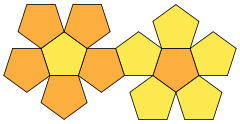A net of a dodecahedron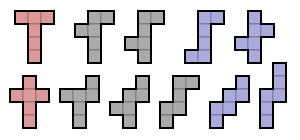The eleven nets of a cube
Various visualizations of the icosahedron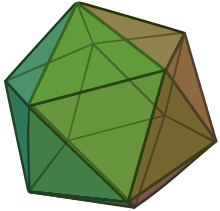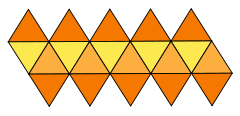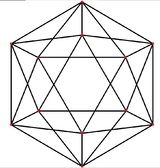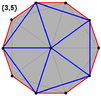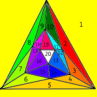In geometry the net of a polyhedron is an arrangement of edge-joined polygons in the plane which can be folded (along edges) to become the faces of the polyhedron. Polyhedral nets are a useful aid to the study of polyhedra and solid geometry in general, as they allow for physical models of polyhedra to be constructed from material such as thin cardboard.

An early instance of polyhedral nets appears in the works of Albrecht Dürer.

## Existence and uniqueness

Many different nets can exist for a given polyhedron, depending on the choices of which edges are joined and which are separated. Conversely, a given net may fold into more than one different convex polyhedron, depending on the angles at which its edges are folded and the choice of which edges to glue together. If a net is given together with a pattern for gluing its edges together, such that each vertex of the resulting shape has positive angular defect and such that the sum of these defects is exactly 4π, then there necessarily exists exactly one polyhedron that can be folded from it; this is Alexandrov's uniqueness theorem. However, the polyhedron formed in this way may have different faces than the ones specified as part of the net: some of the net polygons may have folds across them, and some of the edges between net polygons may remain unfolded. Additionally, the same net may have multiple valid gluing patterns, leading to different folded polyhedra.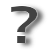Unsolved problem in mathematics:Does every convex polyhedron have a net?(more unsolved problems in mathematics)

In 1975, G. C. Shephard asked whether every convex polyhedron has at least one net. This question, which is also known as Dürer's conjecture, or Dürer's unfolding problem, remains unanswered. There exist non-convex polyhedra that do not have nets, and it is possible to subdivide the faces of every convex polyhedron (for instance along a cut locus) so that the set of subdivided faces has a net. In 2014 Mohammad Ghomi showed that every convex polyhedron admits a net after an affine transformation.

## Shortest path

The shortest path over the surface between two points on the surface of a polyhedron corresponds to a straight line on a suitable net for the subset of faces touched by the path. The net has to be such that the straight line is fully within it, and one may have to consider several nets to see which gives the shortest path. For example, in the case of a cube, if the points are on adjacent faces one candidate for the shortest path is the path crossing the common edge; the shortest path of this kind is found using a net where the two faces are also adjacent. Other candidates for the shortest path are through the surface of a third face adjacent to both (of which there are two), and corresponding nets can be used to find the shortest path in each category.

## Higher-dimensional polytope nets

The geometric concept of a net can be extended to higher dimensions.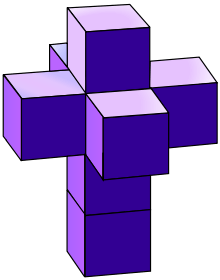Tesseract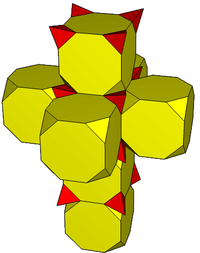Truncated tesseract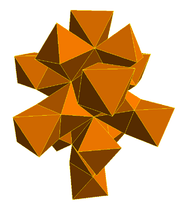24-cell

For example, a net of a 4-polytope, a four-dimensional polytope, is composed of polyhedral cells that are connected by their faces and all occupy the same three-dimensional space, just as the polygon faces of a net of a polyhedron are connected by their edges and all occupy the same plane. The above net of the tesseract, the four-dimensional hypercube, is used prominently in a painting by Salvador Dalí, Crucifixion (Corpus Hypercubus) (1954).

Whether or not every 4-polytope may be cut along the two-dimensional faces shared by its three-dimensional facets, and unfolded into 3D to a single nonoverlapping polyhedron (as in the above unfolding of the tesseract), remains unknown, as does the corresponding question in higher dimensions. However, it is known to be possible for every convex uniform 4-polytope.Wikimedia Commons has media related to Polyhedral net.

4. Demaine, Erik D.; Demaine, Martin L.; Lubiw, Anna; O'Rourke, Joseph (2002), "Enumerating foldings and unfoldings between polygons and polytopes", Graphs and Combinatorics, 18 (1): 93–104, arXiv:cs.CG/0107024, doi:10.1007/s003730200005, MR 1892436
8. Ghomi, Mohammad (2014), "Affine unfoldings of convex polyhedra", Geom. Topol., 18: 3055–3090, arXiv:1305.3231